+关注继续查看

# 1. 路径交叉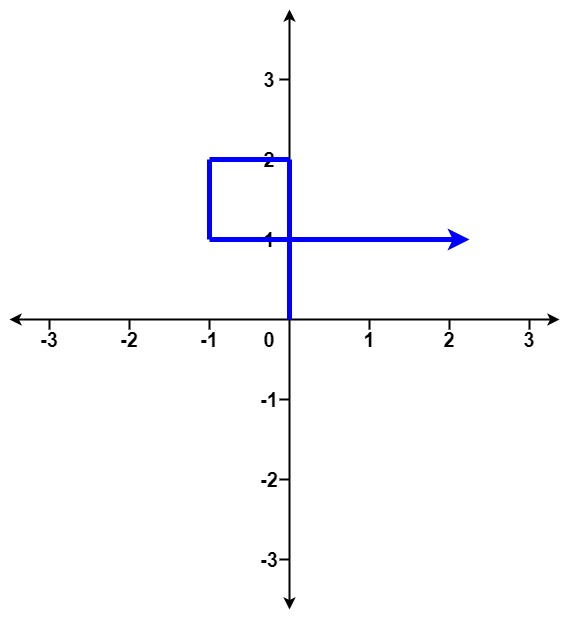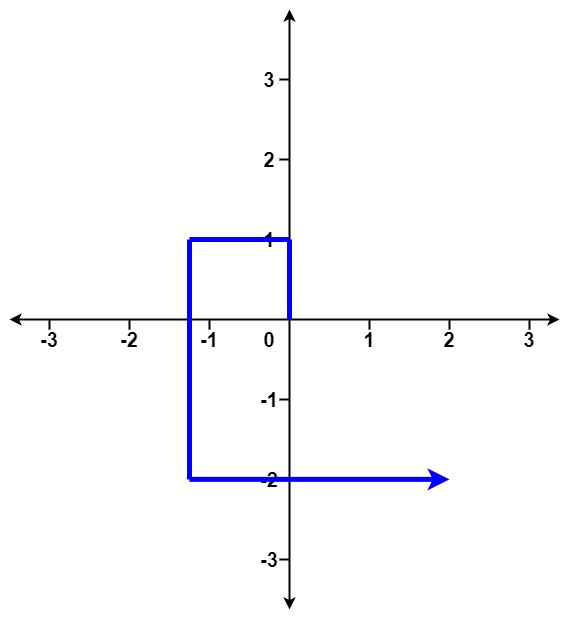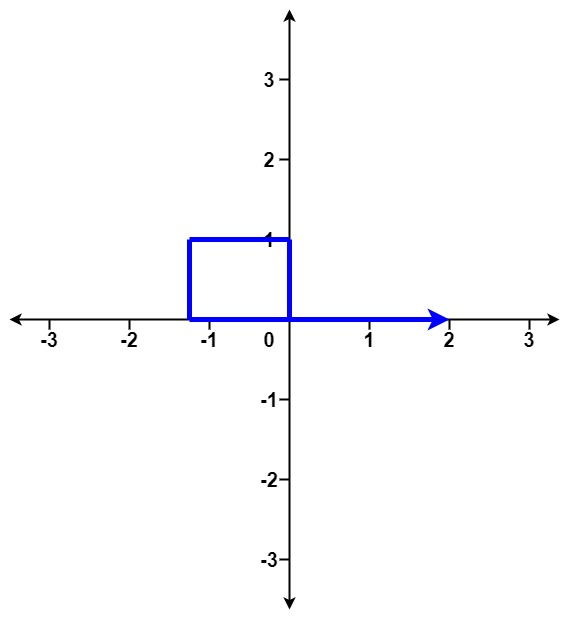• 1 <=?distance.length <= 105
• 1 <=?distance[i] <= 105

class Solution:
def isSelfCrossing(self, x: list) -> bool:
n = len(x)
if n < 3: return False
for i in range(3, n):
if x[i] >= x[i - 2] and x[i - 3] >= x[i - 1]:
return True
if i >= 4 and x[i - 3] == x[i - 1] and x[i] / x[i - 4] >= x[i - 2]:
return True
if i >= 5 and x[i - 3] >= x[i - 1] and x[i - 2] >= x[i - 4] and x[i - 1] + x[i - 5] >= x[i - 3] and x[i] + x[i - 4] >= x[i - 2]:
return True
return False

s = Solution()
distance = [2,1,1,2]
print(s.isSelfCrossing(distance))

distance = [1,2,3,4]
print(s.isSelfCrossing(distance))

distance = [1,1,1,1]
print(s.isSelfCrossing(distance))

True

False

True?

# 2. 缺失的第一个正数

? ?0 <= nums.length <= 300

? ?-2^31 <= nums[i] <= 2^31 - 1

class Solution(object):
def firstMissingPositive(self, nums):
ls = len(nums)
index = 0
while index < ls:
if nums[index] <= 0 or nums[index] > ls or nums[nums[index] - 1] == nums[index]:
index += 1
else:
pos = nums[index] - 1
nums[index], nums[pos] = nums[pos], nums[index]
res = 0
while res < ls and nums[res] == res + 1:
res += 1
return res + 1

# %%
s = Solution()
print(s.firstMissingPositive(nums = [1,2,0]))
print(s.firstMissingPositive(nums = [3,4,-1,1]))
print(s.firstMissingPositive(nums = [7,8,9,11,12]))

3

2

1

# 3. 寻找两个正序数组的中位数

? ?nums1.length == m

? ?nums2.length == n

? ?0 <= m <= 1000

? ?0 <= n <= 1000

? ?1 <= m + n <= 2000

? ?-10^6 <= nums1[i], nums2[i] <= 10^6

import math
from typing import List

class Solution:
def findMedianSortedArrays(self, nums1: List[int],
nums2: List[int]) -> float:
nums1Size = len(nums1)
nums2Size = len(nums2)
na = nums1Size + nums2Size
ns = []
i = 0
j = 0
m = int(math.floor(na / 2 + 1))
while len(ns) < m:
n = None
if i < nums1Size and j < nums2Size:
if nums1[i] < nums2[j]:
n = nums1[i]
i += 1
else:
n = nums2[j]
j += 1
elif i < nums1Size:
n = nums1[i]
i += 1
elif j < nums2Size:
n = nums2[j]
j += 1
ns.append(n)
d = len(ns)
if na % 2 == 1:
return ns[d - 1]
else:
return (ns[d -1] + ns[d - 2]) / 2.0

# %%
s = Solution()
print(s.findMedianSortedArrays([1,3], ))
print(s.findMedianSortedArrays([1,2], [3,4]))
print(s.findMedianSortedArrays([0,0], [0,0]))
print(s.findMedianSortedArrays([], ))
print(s.findMedianSortedArrays(, []))

2

2.5

0.0

1

2

# 附录

## 散列表

Hash table，也叫哈希表，是根据关键码值(Key value)而直接进行访问的数据结构。也就是说，它通过把关键码值映射到表中一个位置来访问记录，以加快查找的速度。这个映射函数叫做散列函数，存放记录的数组叫做散列表。

### 常用方法

· 计算哈希函数所需时间

· 关键字的长度

· 哈希表的大小

· 关键字的分布情况

· 记录的查找频率

1.直接寻址法：

2. 数字分析法：

3. 平方取中法：

4. 折叠法：

5. 随机数法：

6. 除留余数法：Python每日一练(20230518) 螺旋矩阵 I\II\III\IV Spiral Matrix
Python每日一练(20230518) 螺旋矩阵 I\II\III\IV Spiral Matrix
12 0Python每日一练(20230517) 最大连续1的个数 I\II\III
Python每日一练(20230517) 最大连续1的个数 I\II\III
20 0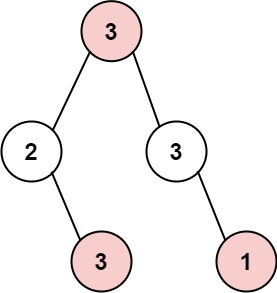Python每日一练(20230516) 打家劫舍 I\II\III\IV HouseRobber
Python每日一练(20230516) 打家劫舍 I\II\III\IV HouseRobber
14 0Python每日一练(20230515) 只出现一次的数字 I\II\III
Python每日一练(20230515) 只出现一次的数字 I\II\III
13 0Python每日一练(20230514) 不同路径 I\II\III UniquePaths
Python每日一练(20230514) 不同路径 I\II\III UniquePaths
15 0Python每日一练(20230513) 粉刷房子 I\II\III Paint House
Python每日一练(20230513) 粉刷房子 I\II\III Paint House
18 0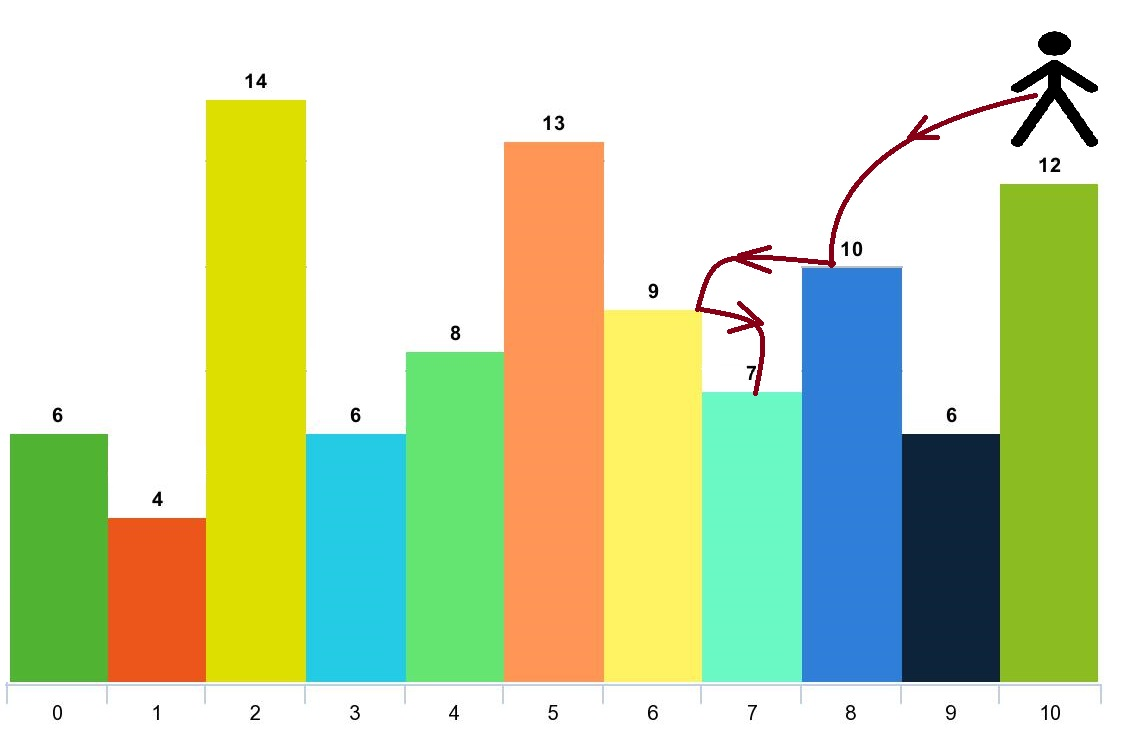Python每日一练(20230512) 跳跃游戏 V\VI\VII
Python每日一练(20230512) 跳跃游戏 V\VI\VII
14 0Python每日一练(20230511) 跳跃游戏 I\II\III\IV
Python每日一练(20230511) 跳跃游戏 I\II\III\IV
18 0Python每日一练(20230510) 石子游戏 VII\VIII\IX
Python每日一练(20230510) 石子游戏 VII\VIII\IX
14 0Python每日一练(20230509) 石子游戏 IV\V\VI
Python每日一练(20230509) 石子游戏 IV\V\VI
14 0
HannYang

Python基础快速入门实战教程378038

Java开发手册（泰山版）